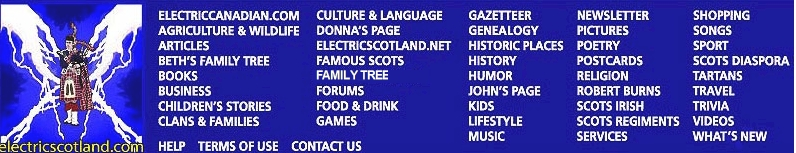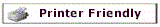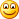Rolfin's Orb Book 1 - Obsidian Word Search Puzzle
 `F T K M F Y Q G U D P G G G Q N L B J O I P E L S C R I B E ` `M G M S L N M E S K R V W Z C R T C B R L H A L Y S N A B D ` `N C F Q F N Q S C E Q Y P X K Z U S Q J U M R I L O M R S K ` `Q N A X Y H W R E A L P I A J E I P O X F T O O R X O B W J ` `D P S L S O W C L O S O P S G D E L B A T I J N C H O R J H ` `Q L G A L J E I F W U T P T I J K F V V Z Z A M H W Z K F F ` `V G N W L I B R X U R V L A R I C O W W B E C H D L Q A R D ` `P E H F Z Q S L L J I W N E B F X C O Q Y T Q A B T F Y F G ` `E C A I S R F T B F E U E O M O N V J B W A N S F W I O C G ` `D O L K V P B N E X D R E E I A M O F G H B M V V V C F B F ` `C A R N K V K A C R O E F D J Q K F F F N L F H T E P S L E ` `K O O S E W Y S E M M C J Y J C D P Y S E F Y H W A Y F S E ` `U A Y T Y E Z B H U P B B M R R O Y X K M V M K Y M Q Q K X ` `K Q O Y U Z P T D G R P X Q M I N D R A Y T O N A J Q V P C ` `C W O F Z T A S I N R W E U U C K E S C H K Z M S N N O J O ` `N O O D H C O L N I T Y H C I C E H Y D R A R T H W O I F X ` `B R R G H K L F V T R T O L E N Y C B S A M N R I H E I W Q ` `B D M M S R P U E T V W E V I U L T V P V L N M I G C A F H ` `A K P U G J S O R I A A Z K R N M L R Q Y Y Z S Y B S I U V ` `U J G F L G V P A N G S O B L Q Z M W N B I N O M K G B E Z ` `L N U F Q L L Z L K O L H U E T O F D C S A I J M D B M U M ` `A A O T H Y A A B Y A L B W Y H X X K P F O T D I T G M K V ` `H M G B V Q R C A S F C E U O A W F F H K R W H J Q V M U E ` `L A C S U M N Z I V S H B D V K J T S H G T V S D X E N Z P ` `B I O U T P Y G W A O J G P N A H K Z L W L S B P A M D I L ` `K G O J D X N E C F R Z M C M A S G H U L D J N N M R F R E ` `I R I A M H P O V P Y C V X C P D N K X O U M P Z G H A R F ` `F H A M W K U D N W G J P N Z Z H D H Y Z R B R E E S T A T ` `Z F E W V C G L Z L F E M C A K P H A B A B U T F D Y H Y B ` `T J M L F P I N G P Y D L N F O L Q L D I O J P P D Q Y Y B ` `ALROY          ANGUS` `ATHDARA        BOOK` `CALLUM         CASTLE` `CATHMORE       COWAN` `DANDELON       DONKEY` `DRAYTON        ELSPET` `FIONA          GAELIC` `GREECE         GYRO` `HYDRA          INVERALBA` `IONMHAS        JIMMY` `JOHNNY         KNITTING` `LOCHDOON       MAIRI` `MCALLISTER     NEEPS` `NIKOLAS        OBSIDIAN` `ORB            SCRIBE` `TABLE`